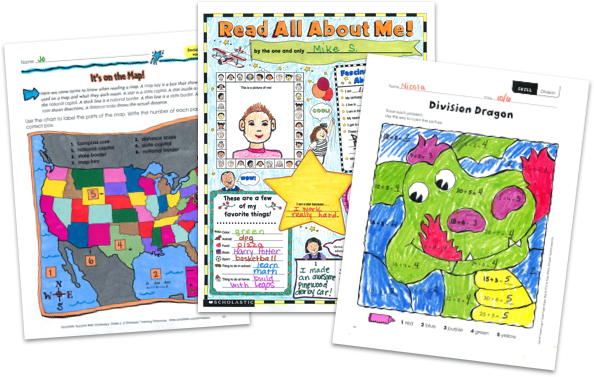MY FILE CABINET# Subtraction Worksheets From Scholastic TeachablesScholastic Teachables (formerly Scholastic Printables) has more than 450 subtraction worksheets for grades Pre-K to 8! Sign up today and get instant online access to printable subtraction worksheets, practice pages, and activities, plus lesson plans, and more to cover all grades and skill levels.

Scholastic Teachables (formerly Scholastic Printables) has more than 450 subtraction worksheets for grades Pre-K to 8! Sign up today and get instant online access to printable subtraction worksheets,  practice pages, and activities, plus lesson plans, and more to cover all grades and skill levels.

## Our Subtraction Worksheets Cover:

• Basic Subtraction
• Charts and Graphs
• Decimals
• Differentiation
• Early Math
• Estimation
• Following Directions
• Fractions
• Life Skills
• Logic and Problem Solving
• Money Math
• Multi-Digit Subtraction
• Number Games
• Number Puzzles
• Real-World Math
• Subtraction With Regrouping
• Subtraction Without Regrouping
• Time and Measurement
• Unit Squares
• Word Problems
• And More!

## Sample Subtraction Worksheets

More than 450 printable subtraction worksheets from Scholastic Teachables cover a range of skills for Pre-K through grade 8! Sign up and you will get access to these as well as a huge selection of other math resources for all grades and skills from Scholastic's more than 30,000 award-winning printables and leveled learning collections in reading and math.

Here's a sample of subtraction worksheets to try in your class—FREE with a 30-day trial or subscription.

## Our Subtraction Worksheets Cover:

• Basic Subtraction
• Charts and Graphs
• Decimals
• Differentiation
• Early Math
• Estimation
• Following Directions
• Fractions
• Life Skills
• Logic and Problem Solving
• Money Math
• Multi-Digit Subtraction
• Number Games
• Number Puzzles
• Real-World Math
• Subtraction With Regrouping
• Subtraction Without Regrouping
• Time and Measurement
• Unit Squares
• Word Problems
• And More!
• Basic Subtraction
• Charts and Graphs
• Decimals
• Differentiation
• Early Math
• Estimation
• Following Directions
• Fractions
• Life Skills
• Logic and Problem Solving
• Money Math
• Multi-Digit Subtraction
• Number Games
• Number Puzzles
• Real-World Math
• Subtraction With Regrouping
• Subtraction Without Regrouping
• Time and Measurement
• Unit Squares
• Word Problems
• And More!

## Sample Subtraction Worksheets

More than 450 printable subtraction worksheets from Scholastic Teachables cover a range of skills for Pre-K through grade 8! Sign up and you will get access to these as well as a huge selection of other math resources for all grades and skills from Scholastic's more than 30,000 award-winning printables and leveled learning collections in reading and math.

Here's a sample of subtraction worksheets to try in your class—FREE with a 30-day trial or subscription.## Additional Alphabet Worksheets (Subscription Required)

Try FREE for 30 days!

If you are not 100% satisfied cancel during the FREE trial and owe nothing. If you are not 100% satisfied cancel during the FREE trial and owe nothing.